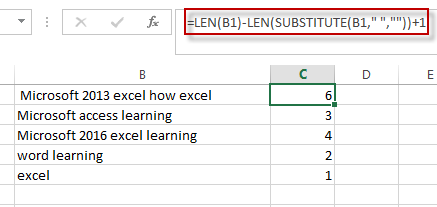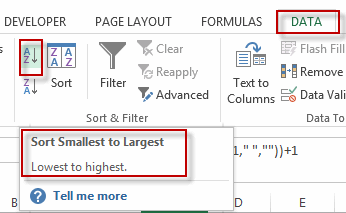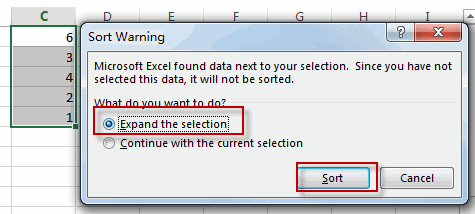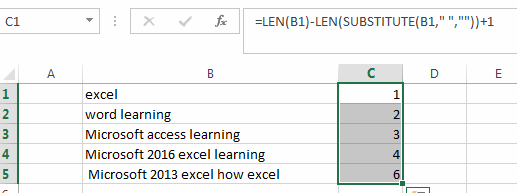# Sort List of Cells by Word Count

This post will guide you how to sort list of cells in excel by word count from the smallest to the biggest. How do I sort on word number of cells with a formula in a column in excel.

Sort List of Cells by Word Count

If you want to sort the list by word count in excel, you need to create an excel formula based on the LEN function and the SUBSTITUTE function.

For example, you want to sort the cells in the range B1:B5, just do the following steps:

#1 type this formula into the formula box of Cell B1, then press Enter key in your keyboard. And drag the AutoFill handler over the other cells to apply this formula to count the word number of cells.

`=LEN(B1)-LEN(SUBSTITUTE(B1," ",""))+1`#2 go to DATA tab, click Sort A to Z command under Sort&Filter group.#3 select Expand the Selection in the Sort Warning dialog. Click Sort button.#4 you will see that the cells have been sorted by word count as below.### Related Functions

• Excel Substitute function
The Excel SUBSTITUTE function replaces a new text string for an old text string in a text string.The syntax of the SUBSTITUTE function is as below:= SUBSTITUTE  (text, old_text, new_text,[instance_num])….
• Excel LEN function
The Excel LEN function returns the length of a text string (the number of characters in a text string).The syntax of the LEN function is as below:= LEN(text)…

Sidebar